## November 2018

### Dipole moment of benzene and its derivatives

Zero dipole moment of the benzene suggested that it has regular hexagonal planer structure and it confirms Kekule’s form.Regular Planer Hexagonal Structure of Benzene having Centre of Symmetry
However, if hydrogen atom is substituted by another atom or group, it acquires polar character.
Examples of such derivative of benzene are C₆H₅Cl, C₆H₅NO₂, C₆H₅OH, etc.
When di-substituted benzene are considered it can be shown that o-isomer will have the highest value of dipole moment then other two isomers. p-derivative has the lowest value while m-derivative has the value between the two.
The values can be calculated using the vector addition principle
μ = m12 + m22 + 2 m1m2 cosθ
provided the group must also lie on the same plane of the benzene ring.

### Di-substituted derivative of benzene: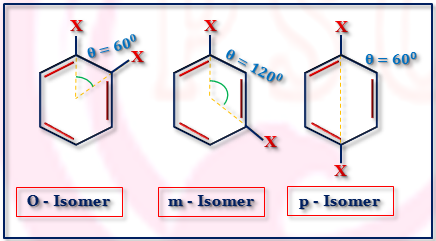Di-substituted derivative of benzene
 μ² = m12 + m22 + 2 m1m2 cosθHere, m1 = m2 = m and θ = 60°Thus, μ² = 2 m²(1 + cos60°)  = 2 m² (1 + 1/2) ∴ μ= √3 m
 μ² = m12 + m22 + 2 m1m2 cosθHere, m1 = m₂ = m and θ = 120°Thus, μ² = 2 m²(1 + cos120°)  = 2 m² (1 - 1/2)∴ μ = m
 μ² = m12 + m22 + 2 m1m₂ cosθHere, m1 = m2= m and θ = 180°Thus, μ² = 2 m²(1 + cos180°)  = 2 m² (1 - 1)∴ μ = 0
Thus μ of o-isomer〉
μ of m-isomer〉μ of p-isomer
(this has been confirmed for C₆H₄Cl₂ and C₆H₄(NO₂)₂.
This determination of dipole moment helps to determine the orientation of the groups in the benzene ring.
• Problem 1:
p - dinitro benzene has μ = 0 but p - dihydroxy benzene μ ≠ 0. Explain.
Through the p-dinitro benzene has μ = 0. p-dihydroxy benzene has dipole moment(μ ≠ 0). This is due to the fact that the two substituted hydroxy group are not on the same plane of the benzene ring but are inclined to the ring.

### (v) Cis and Trans forms of Geometrical Isomerism:

Presence of double bond in C - C link restricts the free rotation and geometrical isomerism develops.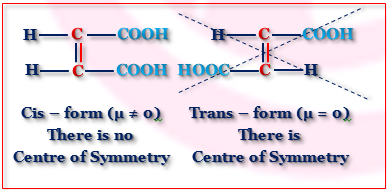Dipole Moment of Cis and Trans Isomers
The Measurement of dipole moment helps to distinguish the two forms of geometrical isomers.

### (vi) Change of Dipole Moment with Temperature:

For molecule CH₂Cl - CH₂Cl, several confirmations are also changed and so dipole moment (μ) of the molecule is also changed.
• Problem 2:
The dipole moment of o-xylene = 0.693 D. Finds the dipole moment of toluene.
For o - xylene the θ = 60° and μ = √3 m. Thus, m = 0.693/√3 = 0.4 D
Again θ = 120° for toluene and dipole moment(μ) of toluene = m.
Thus the dipole moment of toluene is 0.4 D.

### Study polarity of the bond and dipole moments

Evaluation and interpretation of the dipole moment of covalent molecules provide an important tool in the attack of molecular structure. It helps to determine the size and shape of the molecules, spatial arrangements of bonds, bond polarity, residue charge on the atoms of the molecules.

The molecules are composed of partially charged nuclei and negatively charged electrons distributed in space. The structural arrangement of these bonds is different in different molecules.

When the center of gravity of the positive charge due to coincides with the center of gravity of the negative charge due to electrons, the molecules become non-polar.
Hydrogen, carbon dioxide, boron trichloride, phosphorus pentachloride, benzine are the example of non-polar molecules.

When the center of gravity of the positive charge does not coincide with the center of gravity of the negative charge, polarity arises in the molecules and the molecules are called polar.
Hydrogen chloride, water, ammonia, methyl chloride, chlorobenzene are examples of polar molecules.

#### What is the dipole moment of a molecule?

The polarity of a bond in a molecule has quantified a term, called dipole moment (µ).

If +q amount of charge separates at the positive charge center, -q will be accumulated at the negative charge center of the molecule and l is the distance between two centers of the molecule.

∴ Dipole moment, µ = q × l
Non-polar molecules, l=0 and hence µ=0.

Higher the value of µ of a molecule, higher will be its polarity.

#### The polarity of bond in hydrochloric acid

In hydrochloric acid, due to the greater electronegativity of a chlorine atom, the bonding electron pair shifted towards chlorine atom. Thus chlorine atom acquires a small negative charge and hydrogen atom acquires a small positive charge. l is the distance of the charge separation usually taken in bond length.

∴ µ = q × l

The dipole moment is a vector quantity and it has both, magnitude and direction. The direction is represented by an arrow pointing towards the negative end. The length of the arrow is directly proportional to the magnitude of µ.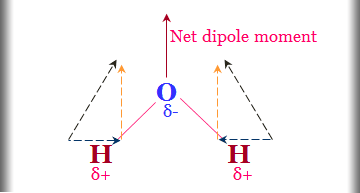Polarity of bond
Problem
P - F, S - F, Cl - F, and F - F which of the following compound has the lowest dipole moment?

The electronegativity difference between the two F atoms is zero. Thus the dipole moment of the fluorine molecule is zero.

#### CGS and SI unit of the dipole moment

In the CGS system, the charge expressed as esu and the length in cm. Thus the unit of dipole moment is esu cm.
The charge in order of 10⁻¹⁰ esu and distance of separation of charge in order of 10⁻⁸ cm.
∴ Order of dipole moment = 10⁻¹⁰ × 10⁻⁸
= 10⁻¹⁸ esu cm.
This magnitude is known as Debye or simply D.

∴ 1 Debye = 10⁻¹⁸ esu cm.

The dipole moment of hydrochloric acid = 1.03 D = 1.03 × 10⁻¹⁸ esu cm.

In the SI system, the charge is expressed in coulomb and length is a meter.

SI unit of dipole moment = coulomb × meter (c × m).

Problem
How can convert 1 Debye to the coulomb meter?

Solution
Dipole moment = q × l.

∴ The dipole moment of a molecule in the CGS unit
= 4.8 × 10⁻¹⁰ × 10⁻⁸ esu cm
= 4.8 D.

The dipole moment of a molecule in the SI unit
1.6 × 10⁻¹⁹ × 10⁻¹⁰ coulombs × meter
= 1.6 × 10⁻³⁰ C × m.

∴ 4.8 Debye = 1.6 ×10⁻³⁰ coulomb meter
or, 1 Debye = (1.6 × 10⁻³⁰)/4.8 coulomb meter
= 3.336 × 10⁻³⁰ coulomb meter.

#### The dimension of the dipole moment

Unit of dipole moment = unit of charge × unit of length.
∴ CGS unit of µ = esu × cm.

Coulomb’s Law, F = q₁q₂/Dr²
∴ (esu)² = dyne × cm² = gm cm sec⁻² × cm²
or, esu = gm1/2 sec⁻¹ cm3/2

∴ Dimension of µ = M1/2 T-1 L5/2

### What is the polarization?

When a non-polar substance placed between two parallel plates and an electric field applied, the field tends to attract the negatively charged electrons towards the positive plate and positive charge towards a negative plate.

Under this condition, there will be the electrical distortion of the molecule and an electric dipole is created. Such distortion in a molecule called the electric polarization.

Polarization, however, disappears as soon as the field is withdrawn and the molecule comes back to its original state.

#### Induced polarization of a molecule

Induced polarization (Pi) and the electric dipole created in the molecule due to the presence of the electric field called induced dipole moment (μi).

The induced dipole moment or simply the induced moment is directly proportional to the strength of the electric field applied(F).
∴ μi ∝ F
When F is low, otherwise, hyperpolarization may occur.
μi = αi F
where αi is proportionality constant called induced polarizability of the molecule.

Induced dipole moment measures the case with which the electronic configuration of the molecule can be distorted by an applied electric field. It may also be defined as the amount of induced moment in the molecule when the unit field strength is applied. The polarizability has the dimension of the volume.

αi = μi/F
∴ Dimension of αi = (esu × cm)/(esu × cm⁻²)
= cm³.

It can also be shown that, αi = r³ where r = radius of the molecule assuming it to have a spherical shape.

For atoms also, distortion occurs when it is placed between the two charged plates. The polarizability of the atom increases with the atomic size, atomic number and the case of ionization energy. Thus atom behaves like a dipole and this dipole moment is induced by the applied electric field.

#### Clausius Mossotti equation from electromagnetic theory

Clausius Mossotti derived from electromagnetic theory, a relation between the polarizability and the dielectric constant of the non-polar medium between two plates.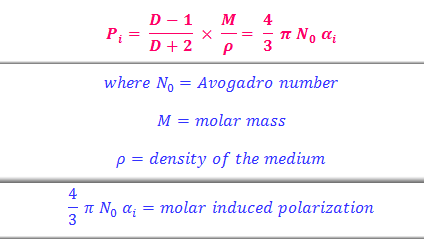Clausius Mossotti equation
Study online for schools and college-level
It gives the distortion produced in the 1 mole of the substance by a unit electric field. Induced polarizability is constant for the molecule and independent of temperature. Hence induced dipole moment is also constant for the molecule and independent of temperature.

D = dielectric constant of the medium = C/C₀
where C = capacitance of the condenser containing the substance and C₀ is the vacuum.
D is the dimensionless quantity and it is unity for vacuum. For other substances, it is greater than unity.

The induced dipole moment of the substance can be calculated by measuring dielectric constant, density (ρ) and knowing the molar mass (M) of the substance.

### Polar bonding molecules - Debye modification

Polar bonding molecules like methyl chloride, water, hydrogen fluoride, etc, molar polarization not constant but decreases with temperature.

Clausius Mossotti equation fails very badly for polar bonding molecules. The reason for the failure was put forward by P Debye.

According to him, when an electric field is applied between two parallel plates containing polar molecules (gas molecules), two effects will occur.

#### Induced polarization of molecules

The field will tend to induced polarization in the molecules and the induced polarization in the molecules.
∴ Pi= (4/3) π N₀ αi

#### Orientation polarization of molecules

The dipolar molecules will be oriented in the field producing a net dipole moment in the direction of the field.
∴ P₀ = (4/3) π N₀ α₀
where α₀ = orientation polarisability.
Debye calculated the value of α₀ = μ²/3KT

Considering the two tendencies that polar molecules tend to orient in the direction of the applied field (applied) and thermal orientation tends to destroy the alignment of the molecules.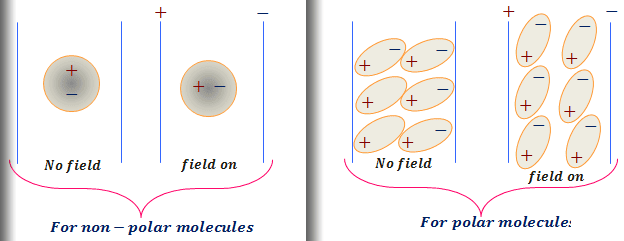Polarization of bond

#### Total polarization of bond

For the polar bonding molecules, the total polarization,
Pt = Pi + P₀Debye equation

#### Temperature dependence on polarizability

A polar bond is fixed and unable to orient in the fixed direction, the orientation polarization has taken zero. This is true for the condensed system where strong intermolecular forces prevent the free rotation of the molecules.

When the temperature is very high tending to infinity.

1/T = 0 and Pt = 0
∴ Pt = Pi

At high temperatures, the polar molecules rotate at such high speed that orientation polarization vanishes.

### Heat capacity definition chemistry

The heat capacity of a substance is defined as the amount of heat required to raise the temperature by one degree.

Usually, the rise in temperature measured in centigrade and heat capacity expressed in calories. Heat capacity depends upon the amount of material in the system.

Heat capacity per gram of substance is called specific heat and per mole called molar heat capacity or simply heat capacity of the substances.

#### Heat capacity at constant volume and constant pressure

The magnitude of heat capacity depends on the pressure and volume, especially in the cases of properties of gases. The temperature of a gm -mole of gas raised by one degree at constant volume. Again in another operation same raise of temperature allowing the volume to very.

The quantity of heat in the two operations would be different. Hence, in mentioning the heat capacity of gas, the condition has to specify.
1. Heat capacity at constant volume denoted by Cv.
2. Heat capacity at constant pressure denoted by Cp.

#### Molar heat capacity definition

The amount of heat required to raise the temperature of one gram of a substance by 1°K called specific heat.
The heat input required to rise by 1°K the temperature of one mole of the substances is called its molar heat capacity or simply heat capacity. The heat capacity denoted by C.

Molar heat capacity = molar mass × specific heat.

Cp = M × cp
Cv = M × cv
where Cp and Cv are the molar heat capacities at constant pressure and constant volume respectively. cp and cv are their specific heats.

Problem
The specific heat at constant pressure and constant volumes are 0.125 and 0.075 cal gm⁻¹ K⁻¹ respectively. Calculate the molecular weight and the atomicity of the gas. Name the gas if possible.

Solution
M = 40 and ⋎ = 1.66(mono-atomic), Ar(Argon).

#### How is specific heat capacity measured?

Let dq heat required to increase the temperature dT for one mole of the substances and its heat capacity = C.
∴ C = dq/dT

dq is a path function and values of dq depend on the actual process followed. We can place certain restrictions to obtain precise values of heat capacity. The usual restrictions are at constant pressure and at constant volume.

Cv = [dq/dT]v and Cp = [dq/dT]p

Definition of internal energy and enthalpy,
dU = dqv and dH = dqp.

∴ Cv = [dq/dT]v = [dU/dT]v
Cp = [dq/dT]p = [dH/dT]p

#### Mechanical work in chemistry

A gas can be heated at constant pressure and constant volume in cylinder fitted with a piston. The gas expands against the piston gives the mechanical work.

In order to attain 1° rise in temperature, the heat supplied should be sufficient to provide the kinetic energy to the molecules and also able to do extra mechanical work.Heat capacity from mechanical work
Cp is some mechanical work is required as additional energy to absorb for lifting piston from volume V₁ to V₂.
Cp - Cv = Mechanical work = PdV
= P(V₂-V₁) = PV₂-PV₁.

If we consider the gas to be an ideal gas
PV = RT.

Cp - Cv = R(T+1) - RT
∴ Cp - Cv = R = 2 calories.

#### Determination of Cv from kinetic energy

Consider the monoatomic gases like argon, helium. If such gases heated at constant volume, the heat supplied will be entirely utilized for increasing the kinetic energy of the translation of the molecules.

Since the monoatomic gas molecules can not any absorption in vibrational or rotational motion.

Volume remaining constant, no energy can be used to do any mechanical work of expansion. Kinetic energy for one-mole gas at the temperature T denoted by E.

E = (3/2)PV = (3/2)RT

Increase of kinetic energy for 1° rise in temperature for monoatomic gas helium, argon

Δ E = (3/2) [(T + 1) - T]R = (3/2) × R
= 3 calories.

The heat supplied at a constant volume equal to the rise in kinetic energy per unit degree rise in temperature.

∴ Cv = ΔE = 3 calories.

For a mole of monoatomic gas the ratio of two heat capacities universally expressed by the symbol ɣ.

ɣ = Cp/Cv = (Cv + R)/Cv
= (3 + 2)/3
= 1.66.

#### The total translational kinetic energy of gas molecules

For polyatomic molecules, the haet supplied used up not only in increasing kinetic energy but also increasing vibrational or rotational energies.

Let x calories of heat used for increasing vibrational or rotational purposes.
∴ Cv = ΔE + x = (3/2)R + x.

Cp = ΔE + mechanical work + x
= (3/2)R + R + x
= (5/2)R + x.
Heat capacity difference remains the same, two calories, as monoatomic gas, but heat capacity ratio

ɣ = {(3/2)R + x}/{(5/2)R + x}
= (5 + x)/(3 + x).

#### Heat capacity of gases from energy

Let molar energy of a gas E.
For monoatomic gas molecules

E = (3/2)RT
∴ Cv = [dE/dT]v = (3/2)R

For polyatomic linear gas molecules

E = (3/2)R + R + (3N - 5)R.
∴ Cv = [dE/dT]v = (3/2)R + R + (3N - 5)R

For polyatomic non-linear gas molecules

E = (3/2)R + R + (3N - 5)R.
∴ Cv = [dE/dT]v = (3/2)R + (3/2)R + (3N - 6)R

### Physical properties of alkenes or olefins

The name olefin coming from the ethylene was called oil-forming gas or olefiant gas. Ethylene forms an oily liquid when treated with chlorine or bromine. The name olefine had granted wide usage and it was decided to compromise and call the series of olefins.
Alkenes or olefins containing two to four carbon atoms are gases, five to seventeen are liquid and eighteen on words solid at room temperature and they burn in air with a luminous flame.
Physical properties of alkenes are similar to those of alkanes since the alkenes are only weak Van der Waals attractive forces.

#### Hydrogenation of alkenes

One way of measuring the stability of an alkene is the determination of its heat of hydrogenation.
CH₂=CH₂ (ΔH = -137 kJ mol⁻¹), MeCH=CH₂ (ΔH = -125.9 kJ mol⁻¹), MeCH₂CH=CH₂(ΔH = -126 kJ mol⁻¹), MeCH₂CH=CH₂(ΔH = -126.8 kJ mol⁻¹), cis- MeCH=CHMe(-119.7 kJ mol⁻¹), trans- MeCH=CHMe(-119.7 kJ mol⁻¹), Me₂C=CH₂(ΔH = -188.8 kJ mol⁻¹).
Since the reaction is exothermic, the smaller ∆H is (numerically), the more stable is the alkene relative to its parent alkane.
Thus, it is only possible to compare the stabilities of different alkenes which produce the same alkane on hydrogenation.

#### Enthalpy of formation of alkenes

This arises from the fact that the enthalpy of formation of alkenes is not purely additive properties; it also depends on steric effects and these tend to vary from molecule to molecule. Since three n- butenes all give the n-butane on reduction.
Thus the order of stabilities
trans but-2-ene 〉 cis but-2-ene 〉 but-1-ene
This order may be explained in terms of steric effect and hyperconjugation.
In but-1-ene, steric repulsion is virtually absent.
In but-2-enes, the two methyl groups in cis isomer being closer together than in the trans isomer, experience greater steric repulsion and consequently the cis form is under greater strain than the trans. Thus steric repulsion destabilizes a molecule.
On the other hand, hyperconjugation stabilizes a molecule, and is small in but-1- ene but much larger in but-2-enes.
Since trans-but-2-ene is the most stable isomer, it follows that hyperconjugation has a greater stabilizing effect then steric repulsion a destabilizing effect.
Problem
Arrange the following Alkenes in order of increasing stability and give your reasons.(i) Me₂C=CH₂, (ii) cis-MeCH=CHMe, (iii) trans-MeCH=CHMe (iv) MeCH₂CH=CH₂
Solution
MeCH₂CH=CH₂(but-1-ene)ㄑcis-MeCH=CHMe(cis but-2-ene)ㄑtrans-MeCH=CHMe(trans but-2-ene) ㄑMe₂C=CH₂(isobutene)
In general, the order of stability of alkenes are R₂C=CR₂ 〉R₂C=CHR 〉R₂C=CH₂ ~ RCH=CHR 〉RCH=CH₂ 〉CH₂=CH₂

### Organic synthesis of alkenes

Owing to the presence of a double bond, the alkenes undergo a large number of addition reactions, but under special conditions, they also undergo substitution reactions.
The high reactivity of the olefinic bond is due to the presence of two π- electrons, and when addition reaction occurs, the trigonal arrangement in the alkene changed to the tetrahedral arrangement in the saturated compound produced.

#### Combustion reactions of alkenes

Alkenes are flammable substances they burn in air with a luminous smoky flame to produce carbon dioxide and water.
2CnH₂n + 3H₂O → 2nCO₂ + 2nH₂O, ΔH = -ve

CH₂=CH₂ + 3O₂ → 2CO₂ + 2H₂O, ΔH = -ve

An addition reaction, organic chemistry, is in its simplest terms an organic reaction where two or more molecules combine to form the larger one and the product is called additive compound.

#### Catalytic hydrogenation of alkenes

Alkenes are readily hydrogenated under pressure in the presence of a catalyst.
Finely divided platinum and palladium are effective at room temperature, nickel on support require a temperature between 200⁰C and 300⁰C, Raney nickel is effective at room temperature and atmospheric pressure.
CH₃CH = CH₂(propene) → CH₂CH₃ - CH₃(propane)

#### Addition of halogens to alkenes

Alkenes from addition compounds with chlorine or bromine.
CH₂ = CH₂(ethylene) + Br₂ → BrCH₂ - CH₂Br(ethylene dibromide)
Halogen addition can take place either by a heterolytic (polar) or a free-radical mechanism.
Halogen addition radially occurs in solution, in the absence of light or peroxides and is catalyzed by inorganic halides as for example aluminum chloride or by polar surfaces. These facts lead to the conclusion that reaction occurs by a polar mechanism.
The free radical mechanism has generally accepted that the addition of halogen to alkenes in the absence of light is polar. Stewart showed that the addition of chlorine to ethylene is accelerated by light and this suggested the free radical mechanism.Organic synthesis of alkenes

Ethylene adds hydrogen bromide to form ethyl bromide.
CH₂=CH₂ + HBr → CH₃⎯CH₂Br

The order of reactivity of the halogen acids is,
hydrogen iodide 〉hydrogen bromide 〉hydrogen chloride 〉hydrogen fluoride
(this is also the order of acid strength).
The conditions for the addition are similar to those for halogens, only the addition of hydrogen fluoride occurs under pressure.
In the case of unsymmetrical alkenes, it is possible for the addition of the halogen acid to take place in two different ways,
Propane might add on hydrogen iodide to form propyl iodide or isopropyl iodide.
CH₃ - CH = CH₂ + HI → CH₃ - CH(H) - CH₂(I)

or it might be,
CH₃ - CH = CH₂ + HI → CH₃ - CH(I) - CH₂(H)
Markownikoff studied many reactions of this kind, and as a result of his work, formulated the following rule.
Online college chemistry courses

#### Markownikoff rule for alkenes

The negative part of the addendum acids on to the carbon atom that is joined to the less number of hydrogen atoms.
In the case of the halogen acids, the halogen atom is the negative part. According to Markownikoff rule, isopropyl halide is obtained.
Markownikoff’s rule is empirical but may be explained theoretically on the basis that the addition occurs by a polar mechanism.
The addition of halogen acid is an electrophilic reaction, the proton adding fast, followed by halide ion. Also, the addition is predominantly trans, and this may explain in terms of the formation of a bridge carbonium ion.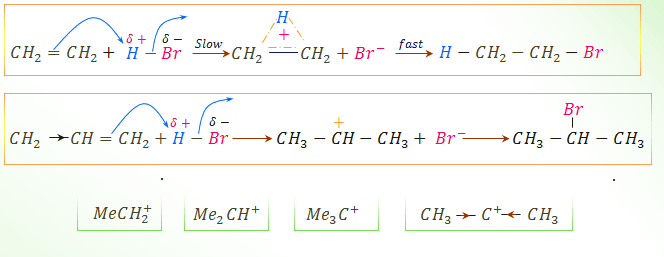The chemical reaction of alkenes
Since the methyl group has a +I effect, the π electrons are displaced towards the terminal carbon atom which, in consequence, acquires a negative charge. Thus, the proton added on to the carbon fastest from the methyl group, and the halide ion then adds to the carbonium ion.
An alternative explanation for Markownikoff's rule is in terms of the stabilities of carbonium ions. Represent as primary, secondary and tertiary carbonium ion.
Problem
Which of the following carbocation is more stable? (i) CH₃CH₂⁺ (ii) CH₃(CH₃)CH⁺ (iii) CH₃(CH₃)C⁺CH₃.
Solution
The number of hydrogen atoms available for hyperconjugation is 3 for (i), 6 for (ii) and 9 for (ii). consequently, (iii) would be expected to be the most stable.
Thus the stability order is
CH₃(CH₃)C⁺CH₃ 〉CH₃(CH₃)CH⁺  〉CH₃CH₂⁺

Name

Email *

Message *